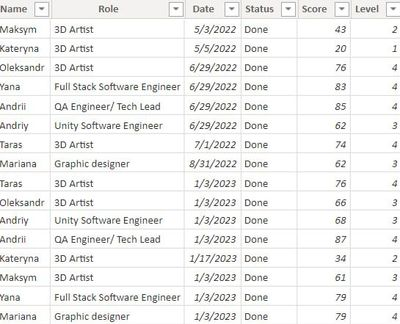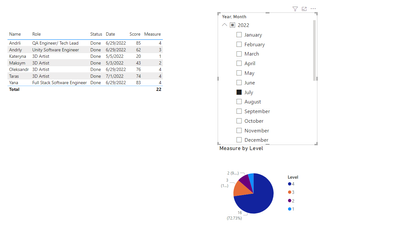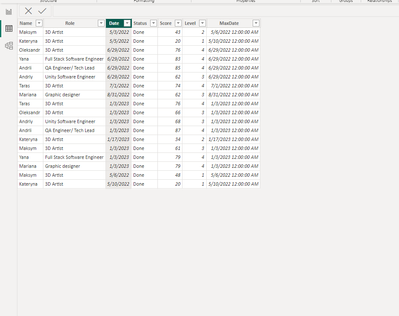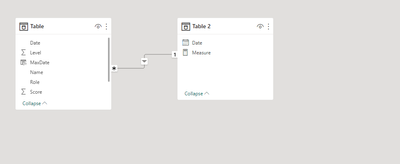cancel
Showing results for
Did you mean:Frequent Visitor

## Current data by month

I have a table in which data on the level of English is entered every few months. If you choose one person, there will be 2-3 entries for that person. It is necessary to write a formula so that when choosing any month, it shows the data of the last entry at that time. And also to be able to create a pie chart where data on the number of people at each level will be indicated and these data will change depending on the selected month1 ACCEPTED SOLUTIONCommunity Support

Try the following suggestion.

1.The date table have no relationship with data table

Then create a column in data table

``Max_level = MAXX(FILTER('Table',[Name]=EARLIER('Table'[Name])&&EOMONTH([Date],0)=EOMONTH(EARLIER('Table'[Date]),0)),[Level]) ``

Then create a new measure

``Measure = CALCULATE(SUM('Table'[Level]),FILTER('Table',EOMONTH([Date],0)<=EOMONTH(MAX('Table 2'[Date]),0)&&[Level]=[Max_level]))``

OutputBest Regards!

Yolo Zhu

If this post helps, then please consider Accept it as the solution to help the other members find it more quickly.

7 REPLIES 7Frequent Visitor

Hi)

This is not exactly what I need.
For example, the data was entered in July, and I choose the month of December. I need in this case to display the latest relevant data on all people. That is, their last levels that were included in the table. And it should be so not only for December, choosing any month should reflect the latest relevant data for that month, taking into account what was entered before it.Community Support

Do you mean to show data for the last level of the selected month and the last level of each month less than that month?

Best Regards!

Yolo ZhuFrequent Visitor

I want that when selecting any month in the filter, it shows me the data that was recorded last. That is, if we choose December, and for the person in July level 3 was recorded, and in September level 4, it will show level 4. If we choose August, it will show level 3 because it was the last one entered.Community Support

Try the following suggestion.

1.The date table have no relationship with data table

Then create a column in data table

``Max_level = MAXX(FILTER('Table',[Name]=EARLIER('Table'[Name])&&EOMONTH([Date],0)=EOMONTH(EARLIER('Table'[Date]),0)),[Level]) ``

Then create a new measure

``Measure = CALCULATE(SUM('Table'[Level]),FILTER('Table',EOMONTH([Date],0)<=EOMONTH(MAX('Table 2'[Date]),0)&&[Level]=[Max_level]))``

OutputBest Regards!

Yolo Zhu

If this post helps, then please consider Accept it as the solution to help the other members find it more quickly.Frequent Visitor

Thank you very much!

And the last question

Is it possible to make it so that it does not display the sum of the levels in the chart, but the number of those levels? for example, 4 levels are 8, 1 level is 1, etc.?Frequent Visitor

And to display not all levels in the graphics. And the last levels are precisely by "Name", because unic Name is less than 16Community Support

You can refer to the following example

Data table1.Create a date table, and create 1:N relationship between two table.

``Table 2 = CALENDAR(DATE(2022,1,1),DATE(2023,1,31))``Then create a nre column in data table

``MaxDate = MAXX(FILTER('Table',[Name]=EARLIER('Table'[Name])&&YEAR([Date])=YEAR(EARLIER('Table'[Date]))&&MONTH([Date])=MONTH(EARLIER('Table'[Date]))),[Date])``

then create a measure in table

``Level = CALCULATE(SUM('Table'[Level]),FILTER('Table',[MaxDate]=[Date]))``

Put the measure to table visual and pie chart

OutputBest Regards!

Yolo Zhu

If this post helps, then please consider Accept it as the solution to help the other members find it more quickly.Announcements#### Exclusive opportunity for Women!

Join us for a free, hands-on Microsoft workshop led by women trainers for women where you will learn how to build a Dashboard in a Day!#### Power Platform Conference-Power BI and Fabric Sessions

Join us Oct 1 - 6 in Las Vegas for the Microsoft Power Platform Conference.Top Solution Authors
Top Kudoed Authors
Users online (2,007)